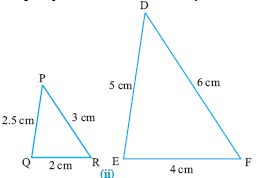Email us to get an instant 20% discount on highly effective K-12 Math & English kwizNET Programs!

#### Online Quiz (WorksheetABCD)

Questions Per Quiz = 2 4 6 8 10

### Geometry2.23 Properties of Similar Triangles

 Congruency and Similarity: Figures that have same shape and size are said to be congruent, whereas figures need to be of the same shape but not necessarily of the same size for them to be similar. Properties of similar triangles Property 1: If in two triangles, angles are equal, then their corresponding sides are proportional and hence the triangles are similar. This property is called AAA postulate. Property 2: If one angle of two triangle is equal to the other and their sides are proportional, then the triangles are similar by SAS postulate. Property 3: If two angles and one side of two triangles are equal, then they are similar by ASA postulate. Example: In triangle ABC, prove that ABC and DEF are similar.Solution:: In triangles ABC and ADE, we have angle A = angle A (common angle) angle B = angle E (90o) Hence triangles ABC and ADE are similar. Directions: Solve the following problems. Also write at least 5 examples of your own.
 Q 1: Find angle EAB75o45o60o Q 2: Find angle DCE80o45o30o Q 3: State if the given pair of triangles are congruent and the criterion used?Answer: Q 4: Find angle AEB45o30o65o Q 5: Find angle DEC45o65o30o Q 6: The perimeters of two similar triangles are 30 and 20 respectively. If one side of the first triangle is 12, determine the corresponding side of the other triangle.8 cm15 cm12 cm Question 7: This question is available to subscribers only! Question 8: This question is available to subscribers only!